NEET  >  Electric Flux

# Electric Flux Notes | Study Physics Class 12 - NEET

## Document Description: Electric Flux for NEET 2022 is part of Physics Class 12 preparation. The notes and questions for Electric Flux have been prepared according to the NEET exam syllabus. Information about Electric Flux covers topics like Analogous with flow of water and concept of flux and Electric Flux Example, for NEET 2022 Exam. Find important definitions, questions, notes, meanings, examples, exercises and tests below for Electric Flux.

Introduction of Electric Flux in English is available as part of our Physics Class 12 for NEET & Electric Flux in Hindi for Physics Class 12 course. Download more important topics related with notes, lectures and mock test series for NEET Exam by signing up for free. NEET: Electric Flux Notes | Study Physics Class 12 - NEET
 Table of contents Analogous with flow of water and concept of flux
 1 Crore+ students have signed up on EduRev. Have you?

Analogous with flow of water and concept of flux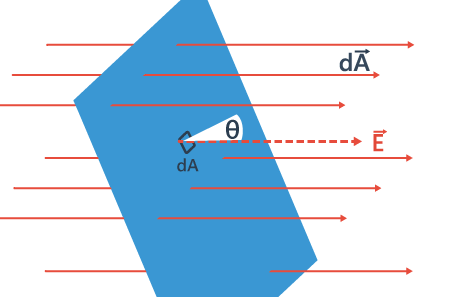Consider flow of a liquid with velocity v, through small flat surface dA, in a direction normal to the surface. The rate of flow of liquid is given by the volume crossing the area per unit time vdA and represents the flux of liquid flowing across the plane. If the normal to the surface is not parallel to the direction of flow of liquid, i.e., to v, but makes an angle θ with it, the projected area in a plane perpendicular to v is vdA cosθ. Therefore the flux going out of the surface d A isV. ñ dA.

For the case of the electric field, we define an analogous quantity and call it electric flux.

We should however note that there is no flow of a physically observable quantity unlike the case of liquid flow. In the picture of electric field lines described above, we saw that the number of field lines crossing a unit area, placed normal to the field at a point is a measure of the strength of electric field at that point.

This means that if we place a small planar element of area ΔA normal to E at a point, the number of field lines crossing it is proportional to E ΔA. Now suppose we tilt the area element by angle θ. Clearly, the number of field lines crossing the area element will be smaller. The projection of the area element normal to E is ΔA cos θ.

Thus, the number of field lines crossing ΔA is proportional to E.ΔA.cosθ. When θ = 90° , field lines will be parallel to ΔA and will not cross it at all (Figure).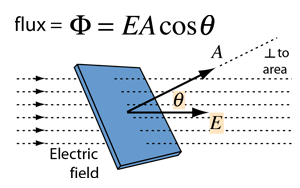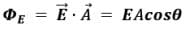where E is the magnitude of the electric field (having units of V/m), A is the area of the surface, and θ is the angle between the electric field lines and the normal (perpendicular) to A.

Note: That that an area element should be treated as a vector. It has a magnitude and also a direction. How to specify the direction of a planar area? Clearly, the normal to the plane specifies the orientation of the plane. Thus, the direction of a planar area vector is along its normal.

But a normal can point in two directions. Which direction do we choose as the direction of the vector associated with the area element?
Conventionally, the vector associated with every area element of a closed surface is taken to be in the direction of the outward normal.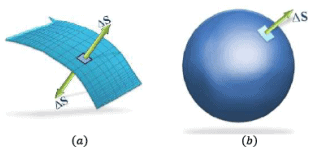In above diagram, note that:

• Represents an open surface, thus, it is irrelevant to define ‘outward’ normal. You can choose any of the two normal at the surface.
• The surface in b is a closed one, it is important to define ‘ outward normal’ as the direction of area vector (as shown in the figure), conventionally.
• For infinitely small area element 𝑑𝐴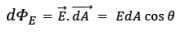And if the Electric field holds different value on different points on the surface, then, we must add up the electric flux from all of them individually,
• For finite number of surfaces (let’ s assume n),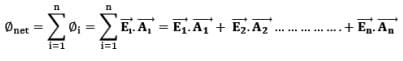• For infinite addition of infinitesimal surfaces i.e. integration,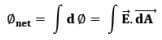• For closed surfaces,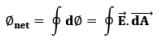Solved Examples
Q.1. An electric field of 500 V/m makes an angle of 30.00 with the surface vector. It has a magnitude of 0.500 m2. Find the electric flux that passes through the surface.
Ans. The electric flux which is passing through the surface is given by the equation as:
ΦE = E.A = EA cos θ
ΦE = (500 V/m) (0.500 m2) cos 30°
ΦE = 217 Vm
Notice: That the unit of electric flux is a volt-time a meter.

Q.2. Consider a uniform electric field E = 3 × 103 î N/C. What is the flux of this field through a square of 10 cm on a side whose plane is parallel to the yz plane?
(a) 30 Nm2/C
(b) 40 Nm2/C
(c) 50 Nm2/C
(d) 60 Nm2/C

Ans. (a)
Solution.
The flux of an electric field is given by,
ϕ = EA ⇒ ϕ = 3 × 10× 0.1 × 0.1 ⇒ ϕ
= 30 Nm2/C
Therefore,  the flux of the field through a square of 10 cm on a side whose plane is parallel to the yz plane is 30 Nm2/C

The document Electric Flux Notes | Study Physics Class 12 - NEET is a part of the NEET Course Physics Class 12.
All you need of NEET at this link: NEET

## Physics Class 12

157 videos|425 docs|213 tests
 Use Code STAYHOME200 and get INR 200 additional OFF

## Physics Class 12

157 videos|425 docs|213 tests

### How to Prepare for NEET

Read our guide to prepare for NEET which is created by Toppers & the best Teachers

Track your progress, build streaks, highlight & save important lessons and more!

,

,

,

,

,

,

,

,

,

,

,

,

,

,

,

,

,

,

,

,

,

;